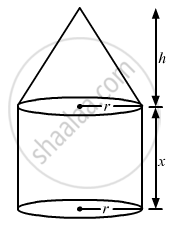Advertisement Remove all ads

# A Solid Consists of a Circular Cylinder Surmounted by a Right Circular Cone. the Height of the Cone is H. If the Total Height of the Solid is 3 Times the Volume of the Cone, Then the Height - Mathematics

MCQ

A solid consists of a circular cylinder surmounted by a right circular cone. The height of the cone is h. If the total height of the solid is 3 times the volume of the cone, then the height of the cylinder is

#### Options

• 2h

• $\frac{3h}{2}$

• $\frac{h}{2}$

• $\frac{2h}{3}$

Advertisement Remove all ads

#### SolutionDisclaimer: In the the question, the statement given is incorrect. Instead of total height of solid being equal to 3 times the volume
of cone, the volume of the total solid should be equal to 3 times the volume of the cone.

Let x be the height of cylinder.

Since, volume of the total solid should be equal to 3 times the volume of the cone,
So,

$\frac{1}{3} \pi r^2 h + \pi r^2 x = 3\left( \frac{1}{3} \pi r^2 h \right)$

$\Rightarrow \frac{1}{3} \pi r^2 h - \pi r^2 h + \pi r^2 x = 0$

$\Rightarrow \pi r^2 x = \frac{2}{3} \pi r^2 h$

$\Rightarrow x = \frac{2}{3}h$

Hence, the height of cylindrical part = (2h)/3

Is there an error in this question or solution?
Advertisement Remove all ads

#### APPEARS IN

RD Sharma Class 10 Maths
Chapter 14 Surface Areas and Volumes
Q 30 | Page 90
Advertisement Remove all ads
Advertisement Remove all ads
Share
Notifications

View all notifications

Forgot password?
Course